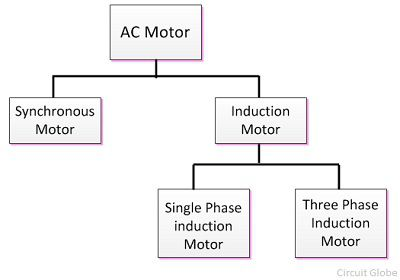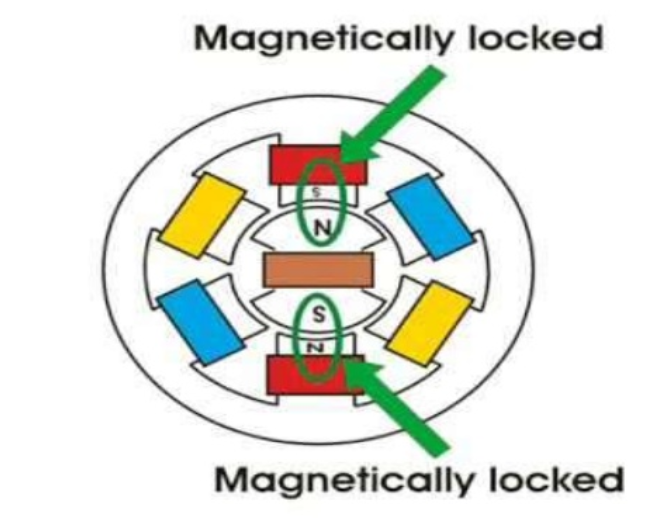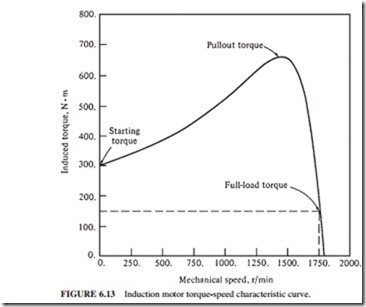This is article is about AC-Motor classes. Firstly, AC motor divided 2 groups synchronous and Indiction (asynchronous) motor.

Synchronous Motor

Firstly, The motor that converts the AC electrical power into mechanical power and is operated only at the synchronous speed is known as a synchronous motor.Syncronous speed can easily be derived as follows,

N=120f/P

where;

N= Synchronous speed

F=Frequency

P= Number of Poles

Speed of synchronous motor can be very accurately controlled. This is the reason why they are suitable for high precision application.

Asynchronous Motor

Secondly, AC motor classes is induction motor or asynchronous motor is an AC electric motor in which the electric current in the rotor needed to produce torque is obtained by electromagnetic induction from the magnetic field of the stator winding.In both asynchronous and synchronous motors, the AC power supplied to the motor’s stator creates a magnetic field that rotates in synchronism with the AC oscillations. In fact, a synchronous motor’s rotor turns at the same rate as the stator field. An induction motor’s rotor rotates at a little bit slower speed than the stator field.